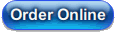# State-Space/DSGE Course (2nd Edition)

The presentation will cover most of Durbin and Koopman's Time Series Analysis by State Space Methods, 2nd Edition, with some additions from Harvey's Forecasting, structural time series and the Kalman filter and West and Harrison's Bayesian Forecasting and Dynamic Models. The textbook replication examples of all of those have many other examples of state-space models done using DLM.

This second edition is greatly expanded compared with the first, with

• new sections on "gap" models with univariate models, bivariate models for gap plus Phillips curve and and non-linear dynamic models
• greatly increased coverage of time-varying coefficients models
• a new section on Dynamic (Multiple) Factor Models
• a detailed discussion of Gibbs sampling methods
• a new section on particle filters
• a new section on Kalman filtering with constraints on the states

### Workbook Contents

(310 pages, 40 examples)

#### 1 Introduction

1.1 State-Space Models
1.2 Kalman Filtering with the Local Linear Model
1.3 Kalman Filtering with the Time-Varying Coefficients Model
1.4 Kalman Smoothing
1.5 Kalman Smoothing with the Local Level Model
1.6 Forecasts and Missing Data
1.7 RATS Tips and Tricks
Example 1.1 Kalman Filter: Nile Data
Example 1.2 Kalman Filter: Drug Sales Data
Example 1.3 Kalman Filter: Time-Varying Coefficients Model
Example 1.4 Kalman Smoothing: Nile Data
Example 1.5 Kalman Smoothing: Estimated Errors
Example 1.6 Missing Data
Example 1.7 Out of Sample Forecasting

#### 2 More States

2.1 Kalman Filter in Matrix Form
2.2 ARMA Processes
2.3 Local Trend Model
2.4 Seasonals
Example 2.1 Local Level vs Local Trend Model

#### 3 Estimating Variances

3.1 The Likelihood Function
3.2 Estimating the Local Level Model
3.3 Estimating the Local Trend Model
3.4 Diagnostics
Example 3.1 Estimating the Local Level Model
Example 3.2 Estimating the Local Trend Model
Example 3.3 Diagnostics

#### 4 Initialization

4.1 Ergodic Solution
4.2 Diffuse Prior
4.3 Mixed Stationary and Non-Stationary Models

#### 5 Practical Examples with a Single Observable

5.1 Basic Structural Models
5.2 Trend plus Stationary Cycle
5.3 Gap Models
5.4 Time-Varying Coefficients in a Linear Model
5.4.1 Fixed Coefficients
5.4.2 Time-varying coefficients
5.4.3 TVC with empirical drift variance
Example 5.1 Airline Data
Example 5.2 Clark Model
Example 5.3 Trend plus Stationary Cycle Model
Example 5.4 Gap Model with Unrestricted Estimates
Example 5.5 Gap Model with Restrictions
Example 5.6 Linear regression with fixed coefficients
Example 5.7 Linear regression with TVC, diagonal drift variance
Example 5.8 Linear regression with TVC, empirical drift variance

#### 6 Practical Examples with Multiple Observables

6.1 Indicator Models
6.2 Multivariate H-P Filter
6.3 Dynamic (Multiple) Factor Models
6.4 Gap Models
Example 6.1 Stock-Watson Indicator Model
Example 6.2 Bivariate H-P Filter
Example 6.4 Dynamic Factor Model, Principal Components
Example 6.5 Bivariate Gap model

#### 7 Interpolation and Distribution

7.1 Linear Model
7.2 Log-Linear Model
7.3 Proportional Denton Method
Example 7.1 Proportional Denton method

#### 8 Simulation Methods

8.1 Conditional Simulation
8.2 Gibbs Sampling
8.2.1 Local Level Model
8.2.2 Time-varying Coefficients
8.2.3 Models with Regressions/Autoregressions
Example 8.1 Simulations
Example 8.2 Gibbs Sampling with Local Level Model
Example 8.3 TVC Model, Gibbs Sampling with Independent Drift Variances
Example 8.4 TVC Model, Gibbs Sampling with Correlated Empirically-Based Drift
Example 8.5 Gibbs Sampling with Linear Regression with AR errors

#### 9 Non-Normal Errors and Non-linear Models

9.1 Stochastic volatity model
9.2 t Distributed Errors
9.3 Non-linearities in the Equations
9.4 Particle filtering
9.5 Restrictions on the States
Example 9.1 Stochastic Volatility Model
Example 9.2 Fat-Tailed Errors
Example 9.3 Non-Linear Kalman Filter
Example 9.4 Particle Filtering
Example 9.5 Constrained Kalman Filter

#### 10 DSGE: Setting Up and Solving Models

10.1 Requirements
10.2 Adapting to different information sets
10.3 Non-linear models
10.4 Unit Roots
10.5 Dynare scripts

#### 11 DSGE: Applications

11.1 Simulations
11.2 Impulse Responses
Example 11.1 DSGE Simulation
Example 11.2 DSGE Impulse Response Functions

#### 12 DSGE: Estimation

12.1 Maximum Likelihood
12.2 Bayesian Methods
12.3 Tips and Tricks
Example 12.1 Maximum Likelihood: Hyperinflation Model
Example 12.2 Maximum Likelihood: Hansen RBC
Example 12.3 Bayesian Estimation: Hyperinflation Model

#### A Probability Distributions

A.1 Uniform
A.2 Univariate Normal
A.3 Beta distribution
A.4 Gamma Distribution
A.5 Inverse Gamma Distribution
A.6 Chi-Squared Distribution
A.7 (Scaled) Inverse Chi-Squared Distribution
A.8 Bernoulli Distribution
A.9 Multivariate Normal
A.10 Wishart Distribution
A.11 Inverse Wishart Distribution